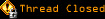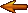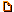# Topic awaiting preservation: Maths... (Page 1 of 1)wrayal
Bipolar (III) Inmate

From: Cranleigh, Surrey, England
Insane since: May 2003posted 07-05-2003 02:07

x^2+2x+1=0
Multiply thru by x
x^3+2x^2+x=0
Factorise:
x(x+1)(x+1)=0
Roots:
0 and -1
Put these through x^2+2x+1=0
Hence my problem.

Wrayal

Go to kimber-ja.demon.co.uk and click on the link to the raytracer!

zyler7952
Nervous Wreck (II) Inmate

From: United Kingdom
Insane since: Jun 2003posted 07-05-2003 02:14

that's quite a bit of a problem you have there. i dont know but i have a friend that might be able to help
will try to help

ok
sorryjdauie
Bipolar (III) Inmate

From: Missoula, MT
Insane since: Jan 2003posted 07-05-2003 04:26

The Equation:
x^2+2x+1=0
is not the same as:
x^3+2x^2+x=0

The first equation has one root:
-1 (double root)
The second equation has two roots:
0, -1 (double root)

What is the problem?

Josh

Tyberius Prime
Paranoid (IV) Mad Scientist with Finglongers

From: Germany
Insane since: Sep 2001posted 07-05-2003 11:35

It's not an informationless transformation, like adding 4 to both sides.

wrayal
Bipolar (III) Inmate

From: Cranleigh, Surrey, England
Insane since: May 2003posted 07-08-2003 23:54

Yes, but adding 4 does not give a new, and incorrect, valyue for x. Mathematically, x^3+2x^2+x=0
and x^2+2x+1=0 are the same!

And BTW, the phrase "multiplying thru by" is used. I am very pedantic, and hate errors like that!

Wrayal

Go to kimber-ja.demon.co.uk and click on the link to the raytracer!

Slime

From: Massachusetts, USA
Insane since: Mar 2000posted 07-09-2003 03:57
quote:
Mathematically, x^3+2x^2+x=0 and x^2+2x+1=0 are the same!

There is something very subtle that is wrong about that statement. The following statement is more accurate:

x^2+2x+1=0
is equivalent to
(x^3+2x^2+x=0) AND (x != 0)

The important thing is that if you multiply both sides of an equation by a number, you can only expect the new equation to be equivalent *if the number was not zero*. If the number was zero, the new equation is not equivalent.

So, if you take x^2+2x+1=0 and multiply both sides by x, you get

(x^3+2x^2+x=0) AND (x != 0)

the roots of this are

(x = 0 OR x = -1) AND (x != 0)

which simplifies to

(x = -1)

So, in other words, what was wrong with your logic was that x^3+2x^2+x=0 and x^2+2x+1=0 are not truly the same. If you want to multiply both sides of an equation by x, and you want the resulting equation to be equivalent, then you must place the extra limitation that x may not be zero.

The reason for this fact is that if you allow x to be zero, it introduces an extraneous root to the equation, as you have seen.

I'd like to find the actual theorem which discusses this formally, but I can't seem to find it online and I don't have any algebra textbooks lying around...

[This message has been edited by Slime (edited 07-09-2003).]